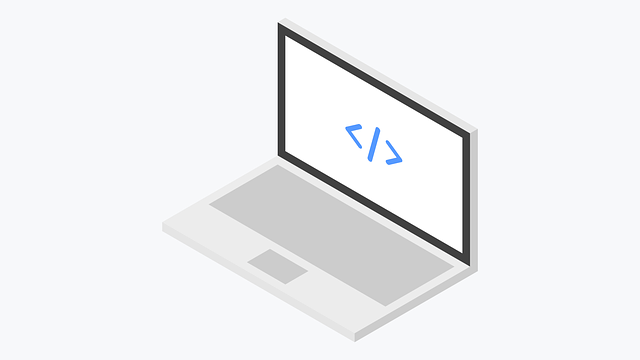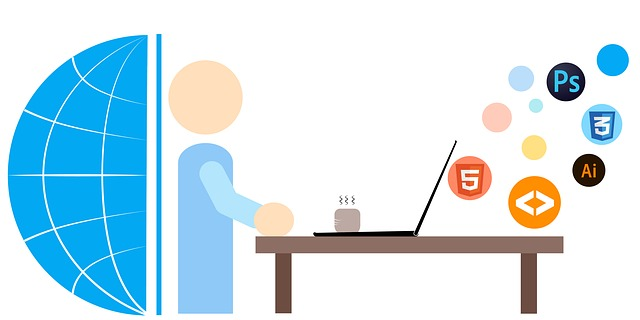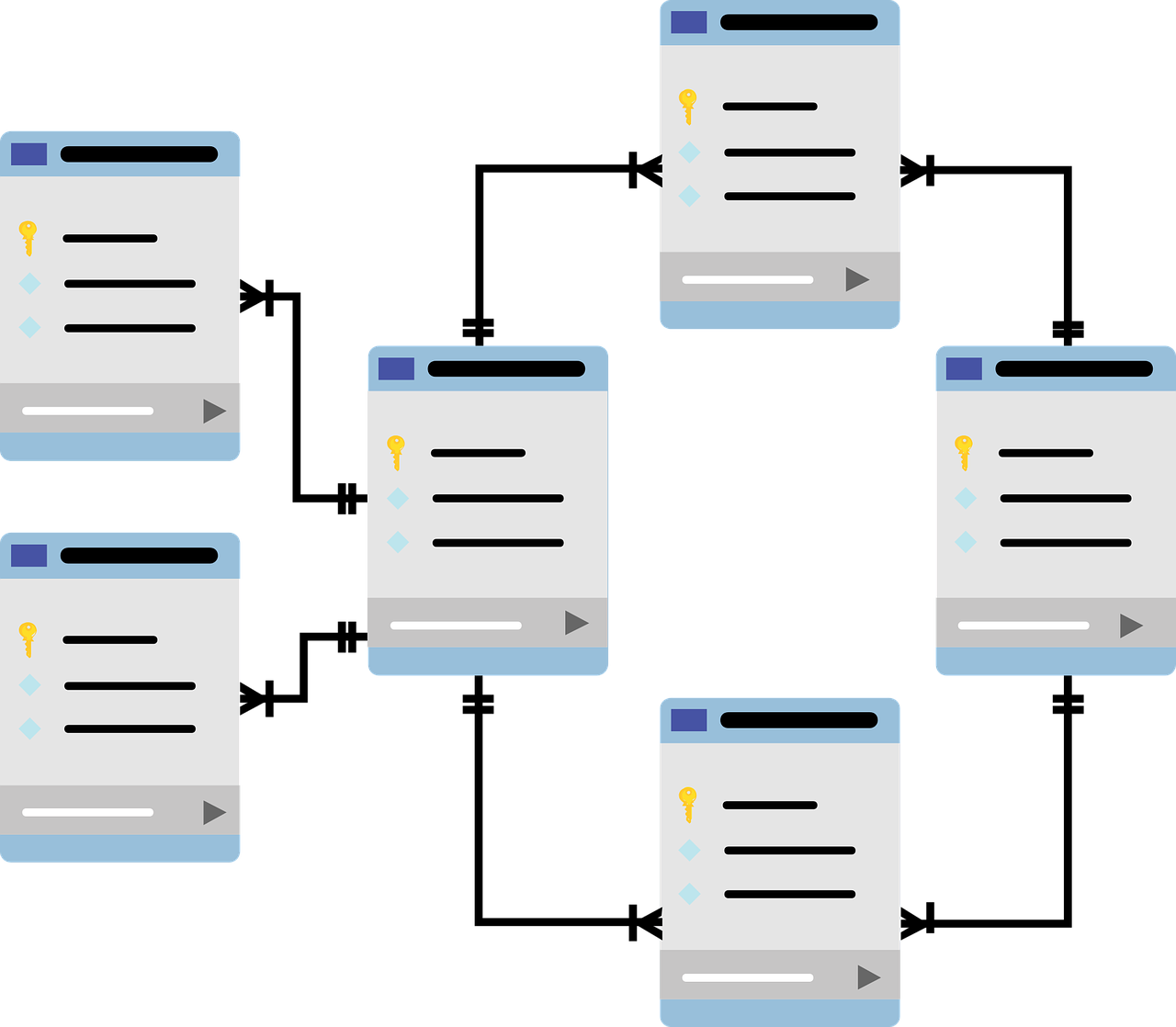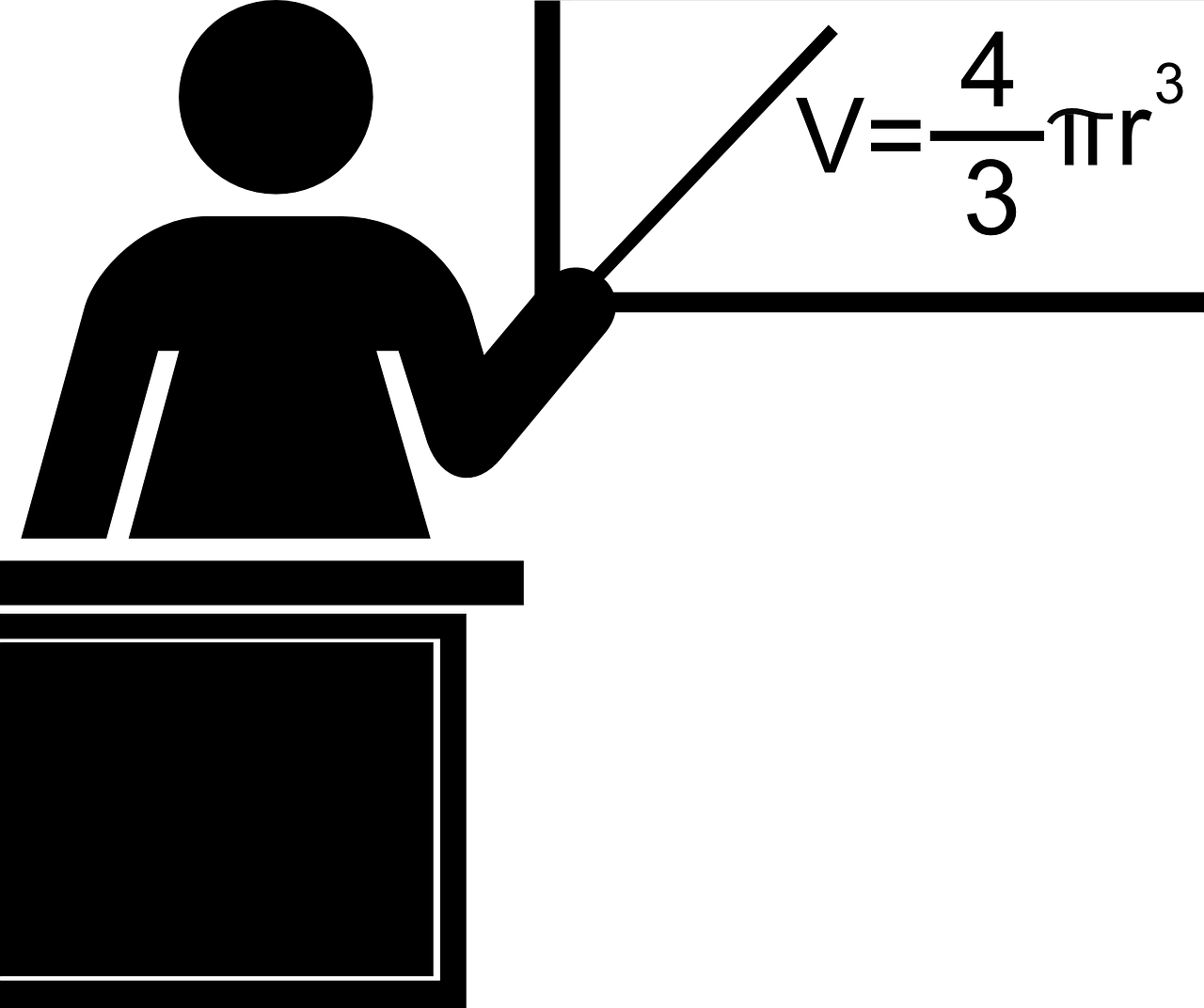LEARN COMPUTER PROGRAMMING

### Learn JavaScript## Learn Programming

Learn most popular programming languages like python, C, C++, Visual Basic 6, etc. Learn web programming with HTML, CSS and JavaScript tutorials.

## Programming Examples

Best way to learn programming languages is to practice as many examples as possible. Learn from our repository of example programs in many popular programming languages.LEARN COMPUTER SCIENCE CONCEPTS

### Computer Networks## Learn Computer Science

Learn computer science to understand various aspects of computer and computer based systems.

Understand the organization, operating systems, database, and algorithms concepts in computer science.

## Learn Mathematics

Mathematics is the foundation of computer science. Learn discrete mathematics, engineering math, and R programming which consists of topics related to computer and data science.

Discrete mathematics is study of finite data whereas engineering math is continues data that are related to physical world.

R programming is used by academics and business houses to perform a statistical analysis of the given data set.